American Journal of Energy Engineering
Volume 3, Issue 2, March 2015, Pages: 16-20

Identifying Defects in the Transmission Lines by Neural Networks

Kherchouche Younes, Savah Houari

Faculty of Electrical Engineering, University Djillali Liabes  , ICEPS (Intelligent Control Electrical Power System) Laboratory, Sidi Bel Abbes, Algeria(K. Younes)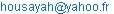(S. Houari)

Kherchouche Younes, Sayah Houari. Identifying Defects in the Transmission Lines by Neural Networks. American Journal of Energy Engineering. Vol. 3, No. 2, 2015, pp. 16-20. doi: 10.11648/j.ajee.20150302.12

Abstract: The paper presents a new technique for the identification of defects in transmission lines using artificial neural networks. The technique uses the amplitude at the fundamental frequency voltage and current signals to one end of the line. A study is conducted to evaluate the performance of the fault identifier.

Keywords: Defects, Identification, Transmission Line, Neural Networks

1. Introduction

A single-phase fault, a tripping and reclosing phase is necessary to maintain system stability.

The identification of defects is used to determine the phase of the faulted transmission line. Different approaches and techniques for identifying defects have been proposed in the literature. The most common technique used is based on the evaluation of signal amplitudes of currents and voltages at the fundamental frequency. The technique is based on the theory of symmetrical components which requires a calculation of Direct amplitudes, Negative and zero sequence components of This technique assumes that the transmission line is ideally transposed, She may have difficulty identifying a two-phase ground fault. Different techniques have been proposed to estimate the amplitudes of the signals, ie using the discrete Fourier transform, Walsh functions , the Kalman filter [2,3], etc.

These techniques use a long computation time and do not have the capacity to adapt dynamically to the operating conditions of the system. The application of neural networks (RN) was too used for identification defects. In  a single neural network is used to identify the default after its occurrence. The inputs to the neural network 5 are samples of the signals of current and voltage at a frequency of 1 khz. The output layer consists of 11 neurons, 10 neurons for each type of defect and a neuron state without blemish. The use of a single neural network with multiple outputs demands large quantity of data in the step of learning and the results are not satisfactory if we consider the variation of network parameters. A single neuron can not identify all fault types correctly. In  the authors use 33 samples per cycle to 2 khz sampled signals as inputs to the neural network. A window of one cycle (or 3 cycles) is used to identify the fault and the fault is identified in 15 ms. In  the authors use 10 neural networks, one neuron for each type of defect. The inputs to the neural networks are the amplitudes of the voltages and currents after fault detection. Each neural network 10 is trained to identify one type of defect. The use of multiple neural networks demands learning each network regardless of where a longer learning time. In ,  and  a single neural network is proposed to identify the fault. The inputs to the neural network are the amplitudes of currents and voltages after the detection of the fault. The neural network has four outputs, three outputs to indicate the faulty phase and an output to indicate if the fault is grounded or not. In this paper, we propose an identifier-based neural defects using the amplitudes of the signals at the fundamental frequency as inputs. Four neural networks are used to identify the defect. Each phase is provided with its specific detector fault based neurons and a neural network is used to identify the implication of the earth.

2. Electric Network Considered

Figure 1 shows the network considered in this study to evaluate the performance of the proposed default identifier. The transmission line of 400 [kv] and a length of 150 [km] from 2 extremities powered by sources GS and GR. The line is represented by parameters distributed by taking into account the dependence of the parameters as a function of frequency. The simulation of the fault line was carried out by the MTLAB software 7.7.0.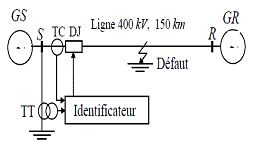Fig. 1. Electrical network considered .

3. Identifier Defects Proposed

Designate by Ia, Ib, Ic the current and Va, Vb, Vc, the voltages of phases a, b and c. Current and zero sequence voltage are appointed by Io and Vo. The identifier of defects (IDEF) proposed consists of four independent networks of neurons: RNA, RNB, RNC and RNG. The first three networks are defect detectors in step (DDP) for detecting the phase (a, b ​​or c) in default and the fourth network is a defect detector to ground (DDT) to indicate the involvement of the ground (G) on default. The outputs of the neural networks RNA, RNB, RNG and RNC are respectively A, B, C, and G (Figure 2).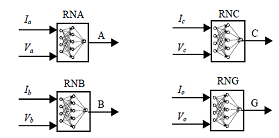Fig. 2. Detectors component IDEF.

4. Input and Output of RNA

The signals calculated at a frequency of 100 khz are processed by a low-pass Butterworth filter of order 4 with a cut off frequency of 300 Hz A sample collection process of 1 khz (20 samples per cycle of 50 Hz ) is applied. This sampling rate is consistent with rates currently used in numerical relays.

A window of data is used as input to the neural network defect detector. The identifier of the default IDEF amplitudes calculated using the fundamental frequency of the discrete Fourier transform DFT  (Ia, Ib, Ic, Io) current and voltage signals (Va, Vb, Vc, Vo) to the place relay as inputs to neural networks (Figure 3).

A series of four consecutive instantaneous amplitudes of the DFT  of each signal (sampled at 1 khz) is selected as input to neural networks to identify the fault. This series is a mobile with a length of 3 ms window. The amplitudes of the DFT  are normalized to reach the input level of the neural networks.

The outputs A, B and C networks are indexed with a value of '1 '(presence of a fault in the corresponding phase) or '0' (situation without default). The output G is indexed with a value of '1 '(default connected to the earth) or '0' (default not related to the earth). The sigmoid transfer function is used in the hidden layer and the output layer. The structures of the neural networks of the IDEF for flaw detectors Phase (DDP) and ground faults (DDT) are summarized in Table 1.

Table 1. Structures flaw detectors phase and earth of the IDEF.

 Detectors to phase faults (DDP) end earth (DDT) Input variables Number of neurons Input layer Hidden layer Output Layer DDP RNA |Ia|,|Va| 8 18 1 RNB |Ib|,|Vb| 8 18 1 RNC |Ic|,|Vc| 8 18 1 DDT RNG |I0|,|V0| 8 18 1

Architectures of neural networks for DDP (NAS) and DDT (RNG) are given in Figures 3 and 4 respectively.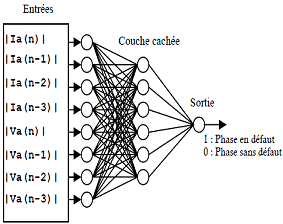Fig. 3. Architectures of RNA network identifier IDEF.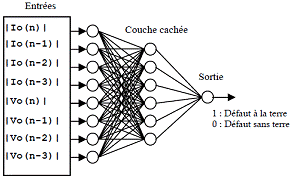Fig. 4. Architectures RNG network identifier IDEF.

5. Tests and Results

After the final learning using Table 2, the neural network identifier IDEF defects are tested using Table 3 with patterns not seen during training using weights and biases the final learning.

Table 2. Parameters for the learning of neural networks.

 Parameter Learning Fault location Lf (km) 3, 40, 80, 120, 147 Angle fault occurred θf (deg) 0, 45, 90 Fault resistance R (Ω) 0, 20, 40 ,100 Power sources (GVA) 9, 20 Voltage source (pu) 0,9, 1,1 Angles sources (load angles in deg.) -20, 0, +20

Table 3. Parameters for testing networks neurons.

 Parameter Learning Fault location Lf (km) 3,4 ,5 ,… 40, 80, 120, 147 Angle fault occurred θf (deg) 0, ,30,60, 90 Fault resistance R (Ω) 0, 5,10,20, 40 ,100 Power sources (GVA) 9, 10,20 Voltage source (pu) 0,9, 1.0,1,1 Angles sources (load angles in deg.) -20, -10,0, 10,+20

We consider the network parameters following: SS = 10 GVA, US = 120° pu, τS = 20 ms et SR= 9 GVA, UR = 0.9pu, τR = 20 ms.

The results in Figure 7a are obtained for a single-phase fault (a-g) 20 km with a fault resistance Rf = 40Ω and t = 30 ms (θ = 600). Figure 7b shows the output of IDEF for phase fault no land (a-b) located 20 km with Rf = 40 Ω and t = 32 ms (θ=900). Figure 7c are obtained for a three-phase fault no land (a-b-c) 100 km t = 28ms (θ=300).Phase A,Phase BPhase C,La terre(g)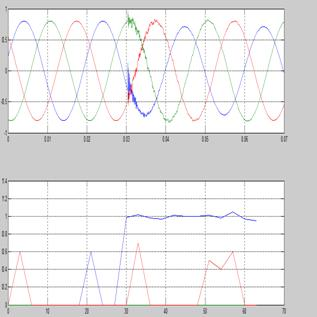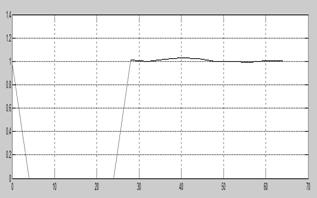Time (ms)

(a)Default (a-g), Lf = 20 km and t = 30 ms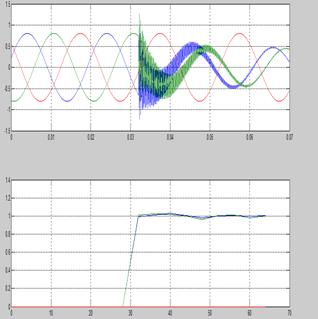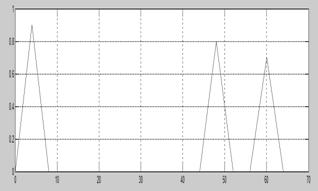Time (ms)

(b)Default (a-b), Lf = 20 km and t = 32ms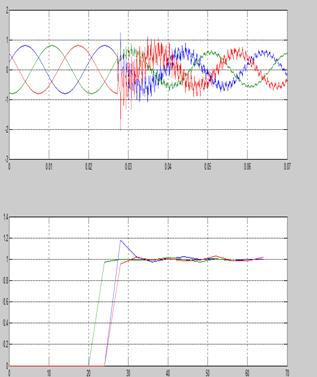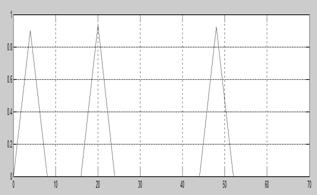Time (ms)

(c)Default (a-b-c), Lf = 100 km and t = 28ms

Fig. 5. Outputs the identifier IDEF.

The results demonstrate the capacity of the fault identifier to pinpoint the type of fault for each test considered .

The response time tr ident of the identifier that is the difference between time toident obtained by the identifier and the actual time of the fault tadef is used as a criterion for evaluating the performance of the identification of the defect. The response time tr ident is defined by: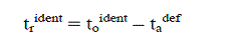(1)

Table 4 gives the values of tr identfor IDEF all fault types and for four values of θf (0 °, 30 °, 60 ° and 90 °) to a 40 km no fault resistance.

Table 4. Results IDEF (tr ident) for a default 40 km no resistance.

 Type of fault Response time tr ident IDEF in ms T=0ms T=28ms T=30ms T=32ms Trident Trident Trident Trident A-g 0 0 2 8 B-g 0 4 2 1 C-g 0 2 3 1 A-b-g 0 4 2 1 A-c-g 0 4 2 1 B-c-g 0 4 2 2 A-b 0 1 4 2 B-c 0 4 2 8 A-c 0 4 2 8 A-b-c 0 4 2 8

Table IV we can see that θ = 0 has its I'DEF gives good results and that the fault occurred angle θf has hardly a rule influence the detection time obtained to ident and the maximum value response time trident is equal to 8 ms obtained for many types of default and angles θf.

Table 5. Results IDEF (tr ident) for a fault at 100 km with resistance.

 Type of fault Response time tr ident IDEF in ms Θf = 0° Θf = 90° Rf = 0 Ω Rf = 40Ω Rf = 100 Ω Rf = 0 Ω Rf = 40 Ω Rf = 100 Ω A-g 1 0 2 4 2 2 B-g 1 2 8 4 2 8 C-g 1 4 4 4 4 4 A-b-g 1 4 1 4 2 2 A-c-g 1 1 1 6 8 2 C-b-g 1 4 4 6 4 4 A-b 1 1 1 8 2 2 A-c 1 1 1 4 2 2 C-b 4 4 4 4 2 2 A-b-c 3 3 3 2 4 4

Table V gives the values tr ident of IDEF for all fault types and θf two values (0 ° and 90 °) for a defect 100 km with three values Rf: 0 Ω, 40 Ω and 100 Ω.

Table 5 shows that the fault resistance has no specific rule of influence on the response time tr ident. The maximum value of tr ident is 8ms obtained for phase-phase faults (a-c-g) for    θf = 90 ° and (b-g) for θf = 90 ° and (a-b) for θf = 90 °.

6. Conclusion

A new approach based neurons is proposed to identify faults in transmission lines in real time. This approach returned in the formation of fast numerical relay. The fault identifier consists of four independent neural networks, each network detects the fault on the phase concerned. The fault identifier uses the amplitudes of the fundamental frequency of the current signals in the place of the relay as the inputs to neural networks. The outputs are used to indicate the type of fault. The results show that the proposed identifier may identify the fault in less than 08 ms matter what the network parameters and fault conditions (fault resistance, fault inception angle, voltage sources, load angles, etc.. .).

Every the test results show that the defect identifier can be used to support a new generation of very fast numerical relays.

References

1. Héctor J.A.F, Ismael D.V. and Ernesto V.M. "Fourier and Walsh digital filtering algorithms forDistance protection", IEEE Trans. On PAS, Vol. 11, No. 1, February, 1996, pp. 457-462.
2. Barros J. And Drake J.M. "Real time fault detection and classification in power systems using Microprocessors", IEE Proc. Gener. Transm. Distrib., Vol. 141, No. 4, July 1994, pp. 315-322.
3. Girgis A.A. and Brown, R.G.: Adaptive Kalman Filtering in Computer Relaying: "Fault Classification Using Voltage Models", IEEE Trans. On Power Apparatus and Systems PAS-104 (1985) no. 5, pp. 1168-1177.
4. Dalstein T. And Kulicke B. "Neural network Approach to fault classification for high speed Protective relaying", IEEE Trans. On Power Delivery, Vol.10 No.2, April 1995 pp. 1002-1011.
5. Kezunovic M., Rikalo I. And Sobajic D. J. "High speed fault detection and classification with neural nets", Electric Power Systems Research, Vol. 34, 1995 pp. 109-116.
6. Poeltl A. And Fröhlich K.: "Two New Methods for Very Fast Type Detection by Means of Parameter Fitting and Artificial Neural Networks", IEEE Transactions on Power Delivery PWRD vol.14 (1999) no. 4, pp. 1269-1275.
7. Pasand M.S and Zadeh H.K. "Transmission line fault detection & phase selection using ANN", International Conference on Power Systems Transients, New Orleans, USA, IPST 2003, cdrom.
8. Aggarwal R. K., Xuan Q. Y., Dunn R.W., Johns A.T. and A. Bennett A.: "A Novel Fault Classification Technique for Double-circuit Lines Based on a Combined Unsupervised/Supervised Neural Network", IEEE Transactions on Power Delivery PWRD vol.14 (1999) no. 4, pp. 1250-1255.
9. Lin W.M, Yang C.D., Lin J.H. and Tsa M.T. "A fault classification method by RBF neural network with OLS learning procedure", IEEE Transactions on Power Delivery vol.16, no. 4, October 2001, pp. 473-477.
10. Logiciel matlab 7.7.0
11. Bouthiba Tahar " Application des réseaux de neurones pour la détection des défauts dans les Lignes de transport ‘’, Conférence Internationale Francophone d’Automatique, ENSEIRB, Bordeaux (France), 30 mai - 1er juin 2006

 Contents 1. 2. 3. 4. 5. 6.
Article ToolsAbstractPDF(347K)